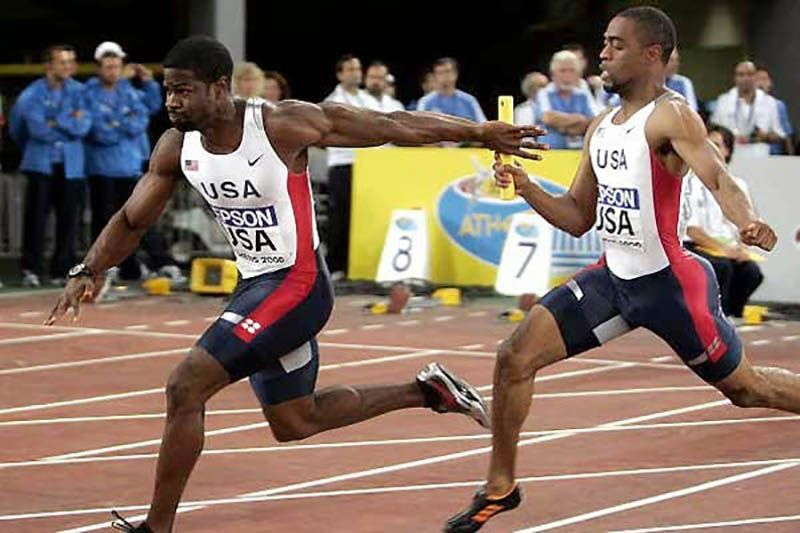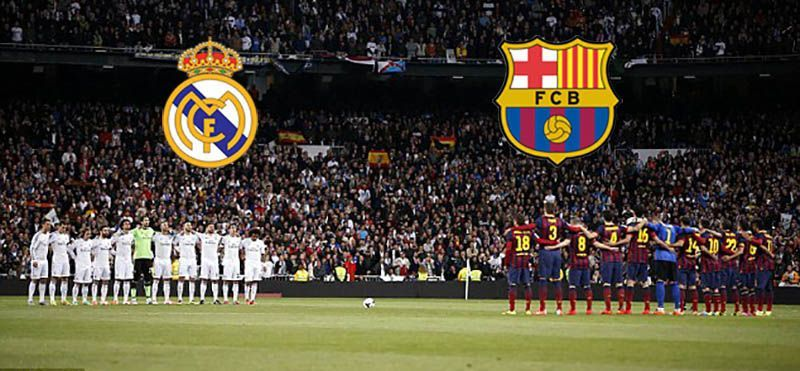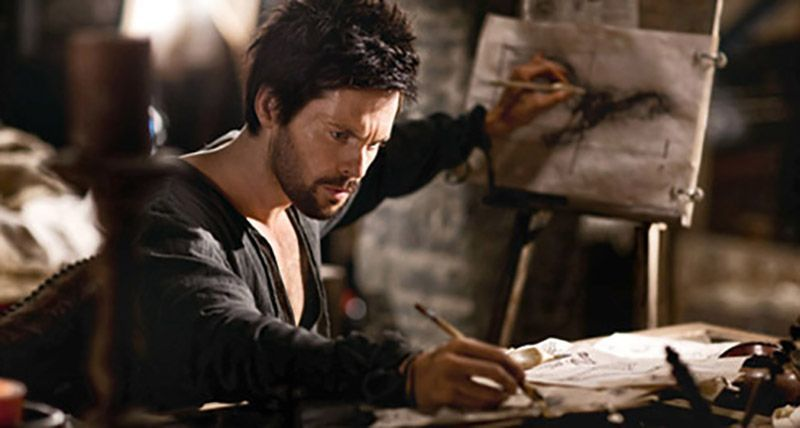# Sequences.

The Relay Race.

The first player stands at the starting point.

The second at 100 meters.

The third at 200 meters.

The fourth at 300 meters.

Sequence - 100, 200, 300, 400, ...Here, the difference is 100.

The value of n = 1, 2, 3, 4, ...

We get the nth term by multiplying the difference with the value of n.

Which can be equal to 100n.

# Example

Tom chased Jerry 4 times on Day 1, 8 times on Day 2 and 12 times on Day 3.

On Day 4 Tom chased 16 times.

# Access All Content

## More from Grade 4 Math# Pictograph

Representing your world in pictures! See how the type and number of a quantity can be mathematically represented pictorially.# Venn Diagram

The easiest way to imagine sets visually. See the power of Venn Diagrams.# Venn Diagram Example

Learn how to use Venn Diagrams to solve real world problems with these interesting examples.